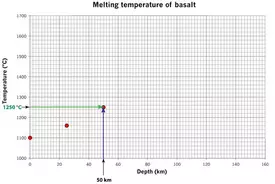# Helping students plot dataAn instructor's guide to Plotting Points

Because many introductory geoscience courses deal with graphs and graphing, we have designed this page to help to remind students how to use the Cartesian coordinate system. This page includes a very basic discussion of how to find the placement of a point on a binary plot.

##What should the student get out of this page?

This page is designed to help students learn the basics of plotting points on a graph. By the time the students complete the module, they should be comfortable with:
• plotting points on an x-y graph (including labeling axes) using a simple algorithm;
• generating a plot of data points from a table of data; and
• recognize instances in which an x-y plot is appropriate.

## Why is it hard for students?

Most students will not struggle with this concept, this is merely an important review for them. However, the students who do have difficulty with graphing may struggle struggle because:
• they are math phobic or avoid math and numbers at all cost. When faced with a table of numbers, these students freeze up or shut down.
• They do not understand the reason for plotting data and have never experienced the need for visualization of a lot of numbers.
• Often in math classes students are asked to generate points from a function in order to determine what a graph of that function looks like; plotting points from data (which rarely is a perfect function!) may be newer for them than we realize.

This page only covers binary plots and generally has only positive numbers plotted on the graphs shown. Although there are instances of ternary plots in the geosciences, we felt that these plots are more common in upper level geoscience courses and were not appropriate for this particular page. We also do not address more difficult non-linear axes such as logarithmic or semi-log axes.

## Instructor resources

Lee Marek at University of Illinois Chicago has a graphing rules page with information for students about how to graph a data set.

Mathworld has a complex definition of graph with links to multiple types of graphs.

The Center for Support of Teaching and Learning at Syracuse University has two tutorials for students that covers the visual display of information on graphs.

There are also several activities and exercises available on the Teach the Earth part of SERC.
• Plotting Data

A module at Starting Point designed to give faculty help with teaching students about plotting data on graphs. Each link gives some information about the important points for plotting points on graphs.
• Describing Graphs

Starting Point has this module about describing graphs with a number of good links for both faculty and students. As with the Plotting module, there are a number of useful links to web pages that address student difficulty with describing graphs.
• Activities designed to increase and develop quantitative skills

A number of the activities posted on the Quantitative Skills in the Geosciences site are designed to help students learn about graphing in a geoscience context. Some of these activities are designed for upper level students.
• Introductory level activities involving Excel

There are dozens of activities that involve the use of Excel that are available as a part of SERC's Starting Point. Each of these activities is downloadable and ready to use.
• ConcepTests involving intepretation of graphs

There are a number of examples of ConcepTests that can be used to break up lecture in a large classroom. Each of these ConcepTests involves the interpretation of graphs appropriate to introductory level geoscience.